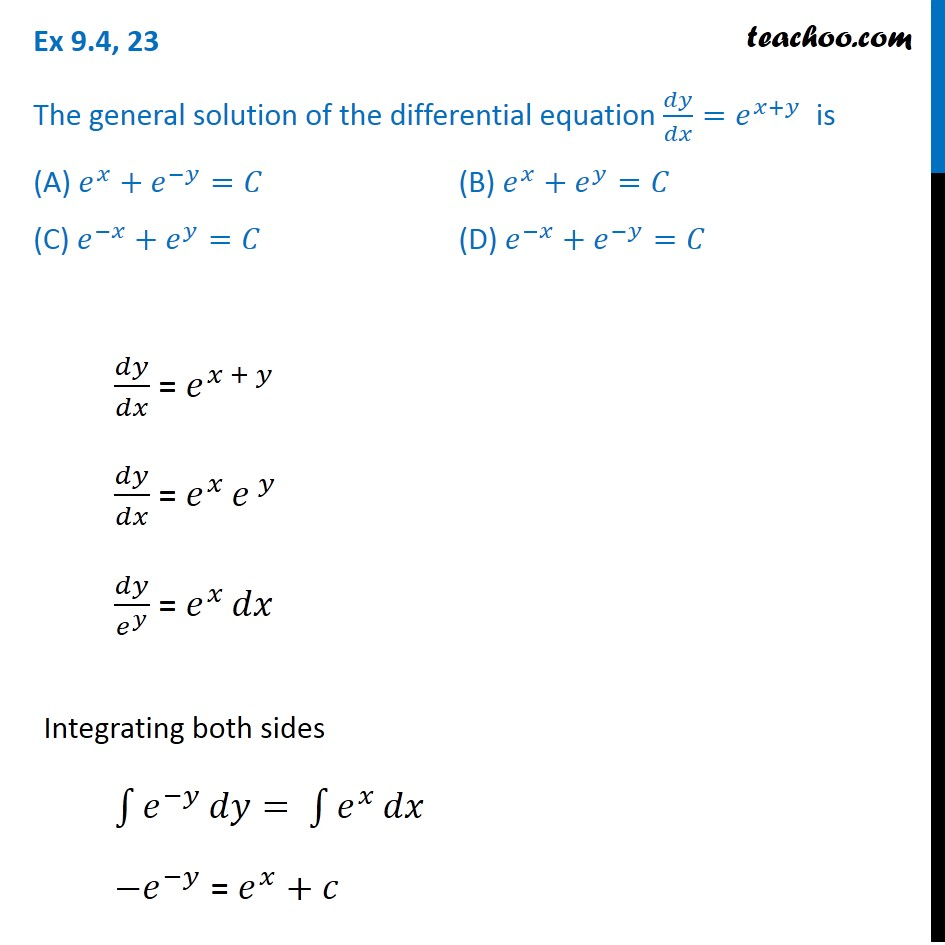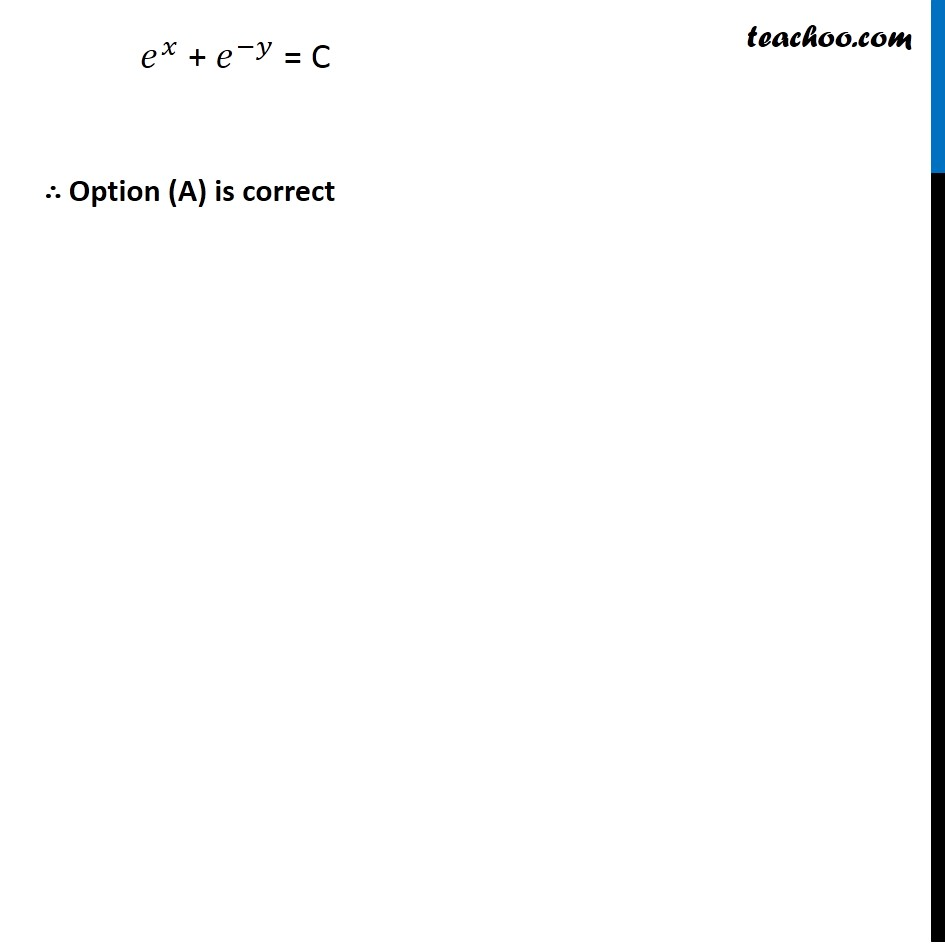Ex 9.3

Chapter 9 Class 12 Differential Equations
Serial order wiseLearn in your speed, with individual attention - Teachoo Maths 1-on-1 Class

### Transcript

Ex 9.3, 23 The general solution of the differential equation 𝑑𝑦/𝑑𝑥=𝑒^(𝑥+𝑦) is (A) 𝑒^𝑥+𝑒^(−𝑦)=𝐶 (B) 𝑒^𝑥+𝑒^𝑦=𝐶 (C) 𝑒^(−𝑥)+𝑒^𝑦=𝐶 (D) 𝑒^(−𝑥)+𝑒^(−𝑦)=𝐶 𝑑𝑦/𝑑𝑥 = 𝑒^(𝑥 + 𝑦) 𝑑𝑦/𝑑𝑥 = 𝑒^(𝑥 ) 𝑒^( 𝑦) 𝑑𝑦/𝑒^𝑦 = 𝑒^(𝑥 ) 𝑑𝑥 Integrating both sides ∫1▒𝑒^(−𝑦) 𝑑𝑦= ∫1▒𝑒^𝑥 𝑑𝑥 〖−𝑒〗^(−𝑦) = 𝑒^𝑥+𝑐 𝑒^𝑥 + 𝑒^(−𝑦) = C ∴ Option (A) is correct.